Engineering ToolBox - Resources, Tools and Basic Information for Engineering and Design of Technical Applications!

# Air - Specific Heat vs. Temperature at Constant Pressure

## Online calculator with figures and tables showing specific heat (Cp and Cv) of dry air vs. temperature and pressure. SI and imperial units.

Specific heat (C) is the amount of heat required to change the temperature of a mass unit of a substance by one degree.

• Isobaric specific heat (Cp) is used for air in a constant pressure (ΔP = 0) system.
• Isochoric specific heat (Cv) is used for air in a constant-volume, (= isovolumetric or isometric) closed system.

Note! At normal atmospheric pressure of 1.013 bar - the specific heat of dry air - CP and CV - will vary with temperature. This may influence on the accuracy of air conditioning and industrial air handling process calculations. When calculating mass and volume flow of air in heated or cooled systems with high accuracy - the specific heat (= heat capacity) should be corrected according values in the figures and table below, or found by use of the calculator.

• For ordinary calculations - a value of specific heat cp = 1.0 kJ/kg K (equal to kJ/kg oC) or 0.24 Btu(IT)/lb °F - is normally accurate enough
• For higher accuracy - a value of Cp = 1.006 kJ/kg K (equal to kJ/kg oC) or 0.2403 Btu(IT)/lb °F  - is better

### Online Air Specific Heat Calculator

The calculator below can be used to estimate the specific heat of air at constant volum or constant pressure and at given temperature and pressure.
The output heat capacity is given as kJ/(kmol*K), kJ/(kg*K), kWh/(kg*K), kcal/(kg*K), Btu(IT)/(mol*°R) and Btu(IT)/(lbm*°R)

Temperature

Choose the actual unit of temperature: °C  °F  °R
Choose the actual pressure:  1 bara / 14.5 psia; 10 bara / 145 psia; 50 bara / 725 psia; 100 bara / 1450 psia;

as well as Specific heat  of Ammonia, Butane, Carbon dioxide, Carbon monoxide, Ethane, Ethanol, Ethylene, Hydrogen, Methane, Methanol, Nitrogen, Oxygen, Propane and Water.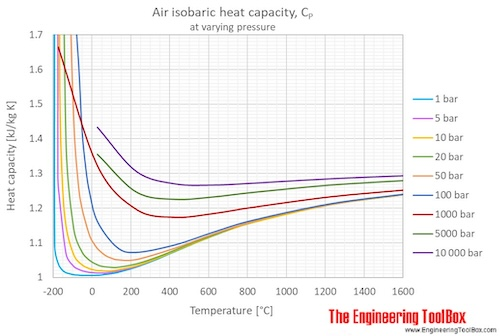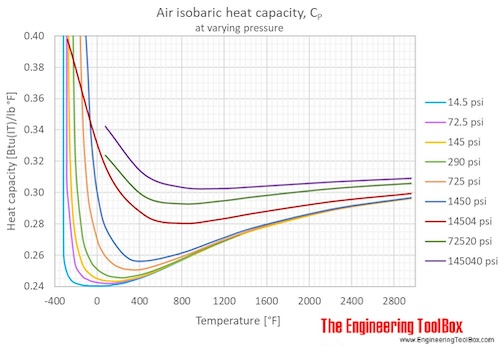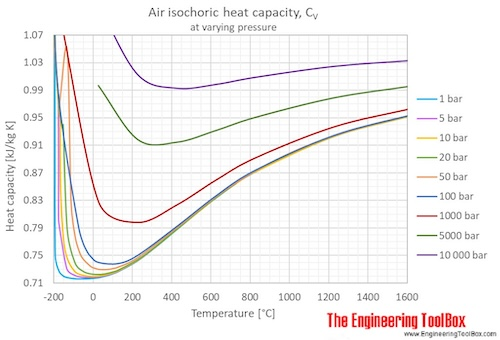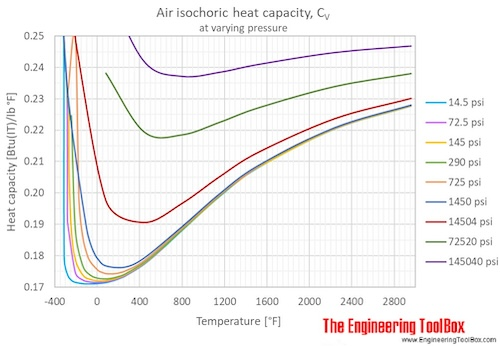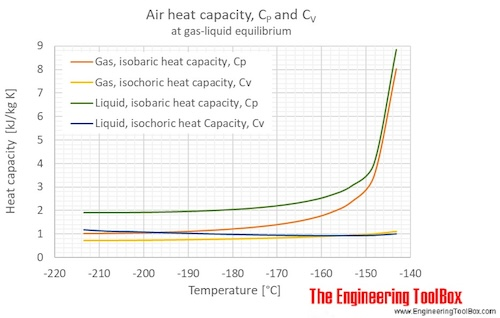Specific heat of air at 1 bar (=0.1 mPa = 14.5 psi):

For full table with Isobaric heat capacity - rotate the screen!

 Temperature Isochoric specific heat (Cv) Isobaric specific heat (Cp) Cp/Cv [K] [°C] [°F] [kJ/mol K] [kJ/kg K] [kWh/(kg K)] [kcal(IT)/(kg K)][Btu(IT)/lb °F] [kcal(IT)/(lb °F)] [kJ/mol K] [kJ/kg K] [(kW h)/(kg K)] [kcal(IT)/(kg K)][Btu(IT)/lb °F] [kcal(IT)/(lb °F)] [-] 60 -213 -352 0.03398 1.173 0.0003258 0.2802 0.2287 0.05506 1.901 0.000528 0.45405 0.37071 1.621 78.79 -194 -318 0.03044 1.051 0.0002919 0.2510 0.2050 0.05599 1.933 0.000537 0.46169 0.37695 1.839 81.61 -192 -313 0.02172 0.7500 0.0002083 0.1791 0.1463 0.03154 1.089 0.000303 0.26010 0.21237 1.452 100 -173 -280 0.02109 0.7280 0.0002022 0.1739 0.1420 0.03012 1.040 0.000289 0.24833 0.20276 1.428 120 -153 -244 0.02093 0.7225 0.0002007 0.1726 0.1406 0.02953 1.022 0.000283 0.24350 0.19930 1.415 140 -133 -208 0.02081 0.7184 0.0001996 0.1716 0.1401 0.02937 1.014 0.000282 0.24219 0.19774 1.411 160 -113 -172 0.02077 0.7172 0.0001992 0.1713 0.1399 0.02928 1.011 0.000281 0.24147 0.19716 1.410 180 -93.2 -136 0.02076 0.7166 0.0001991 0.1712 0.1397 0.02920 1.008 0.000280 0.24076 0.19657 1.407 200 -73.2 -99.7 0.02075 0.7163 0.0001990 0.1711 0.1397 0.02917 1.007 0.000280 0.24052 0.19638 1.406 220 -53.2 -63.7 0.02075 0.7163 0.0001990 0.1711 0.1397 0.02914 1.006 0.000279 0.24028 0.19618 1.404 240 -33.2 -27.7 0.02075 0.7164 0.0001990 0.1711 0.1397 0.02914 1.006 0.000279 0.24028 0.19618 1.404 260 -13.2 8.3 0.02076 0.7168 0.0001991 0.1712 0.1398 0.02914 1.006 0.000279 0.24028 0.19618 1.403 273.2 0.0 32.0 0.02077 0.7171 0.0001992 0.1713 0.1398 0.02914 1.006 0.000279 0.24028 0.19618 1.403 280 6.9 44.3 0.02078 0.7173 0.0001993 0.1713 0.1399 0.02914 1.006 0.000279 0.24028 0.19618 1.402 288.7 15.6 60.0 0.02078 0.7175 0.0001993 0.1714 0.1399 0.02914 1.006 0.000279 0.24030 0.19620 1.402 300 26.9 80.3 0.02080 0.7180 0.0001994 0.1715 0.1400 0.02915 1.006 0.000280 0.24036 0.19625 1.402 320 46.9 116 0.02083 0.7192 0.0001998 0.1718 0.1403 0.02917 1.007 0.000280 0.24052 0.19638 1.400 340 66.9 152 0.02087 0.7206 0.0002002 0.1721 0.1405 0.02923 1.009 0.000280 0.24100 0.19677 1.400 360 86.9 188 0.02092 0.7223 0.0002006 0.1725 0.1409 0.02926 1.010 0.000281 0.24123 0.19696 1.398 380 107 224 0.02098 0.7243 0.0002012 0.1730 0.1412 0.02931 1.012 0.000281 0.24171 0.19735 1.397 400 127 260 0.02105 0.7266 0.0002018 0.1735 0.1417 0.02937 1.014 0.000282 0.24219 0.19774 1.396 500 227 440 0.02150 0.7424 0.0002062 0.1773 0.1448 0.02983 1.030 0.000286 0.24597 0.20083 1.387 600 327 620 0.02213 0.7641 0.0002123 0.1825 0.1490 0.03044 1.051 0.000292 0.25103 0.20496 1.375 700 427 800 0.02282 0.7877 0.0002188 0.1881 0.1536 0.03114 1.075 0.000299 0.25675 0.20963 1.365 800 527 980 0.02351 0.8117 0.0002255 0.1939 0.1583 0.03183 1.099 0.000305 0.26249 0.21432 1.354 900 627 1160 0.02415 0.8338 0.0002316 0.1991 0.1626 0.03247 1.121 0.000311 0.26772 0.21858 1.344 1100 827 1520 0.02525 0.8716 0.0002421 0.2082 0.1700 0.03356 1.159 0.000322 0.27675 0.22596 1.329 1500 1227 2240 0.02673 0.9230 0.0002564 0.2204 0.1800 0.03505 1.210 0.000336 0.28901 0.23597 1.311 1900 1627 2960 0.02762 0.9535 0.0002649 0.2277 0.1859 0.03593 1.241 0.000345 0.29631 0.24193 1.301

Unit conversion:

Specific heat unit converter

british thermal unit(International table) = [Btu(IT)], degree celcius = [°C], degree fahrenheit = [°F], kelvin = [K], degree rankin = [°R], joule = [J], kilocalorie(International table) = [kcal(IT)], kilogram = [kg], kilojoule = [kJ], kilowatthour = [kWh], mole = [mol], pound =[lb]

K in the units can be replaced by °C, and vise versa. °R in the units can be replaced by °F, and vise versa.

• 1 Btu/(lb °F) = 1 Btu/(lb °R) = 1 kcal(IT)/(kg °C) = 1 kcal(IT)/(kg K) = 4186.8 J/(kg K) = 0.81647 kcal(IT)/(lb °F) = 1.163x10-3 kWh/(kg K)
• 1 J/(kg K) = 1 J/(kg °C) = 2.3885x10-4 kcal(IT)/(kg oC) = 2.3885x10-4 Btu/(lb °F) = 1.9501x10-4 kcal(IT)/(lb °F)
• 1 kcal(IT)/(kg °C) = 1 Btu/(lb °F) = 4186.8 J/(kg K) = 0.81647 kcal(IT)/(lb °F) = 1.163x10-3 kWh/(kg K)
• 1 kcal(IT)/(lb °F) = 1.2248 Btu/(lb °F) = 1.2248 kcal(IT)/(kg °C) = 5127.9 J/(kg K)
• 1 kJ/(kg K) = 1 kJ/(kg °C) = 1000 J/(kg K) = 1000 J/(kg °C) = 0.23885 kcal(IT)/(kg °C) = 0.23885 Btu/(lb °F) = 0.19501 kcal(IT)/(lb °F) = 2.7778x10-4 kWh/(kg K)
• 1 kWh/(kg K) = 0.85985 kcal(IT)/(kg °C) = 0.85985 Btu/(lb °F) = 3.6 kJ/(kg K)
• 1 mol of air = 28.96546 g

## Related Topics

• ### Air Psychrometrics

Moist and humid air - psychrometric charts, Mollier diagrams, air-condition temperatures and absolute and relative humidity and moisture content.
• ### Fluid Mechanics

The study of fluids - liquids and gases. Involving velocity, pressure, density and temperature as functions of space and time.
• ### Gases and Compressed Air

Air, LNG, LPG and other common gas properties, pipeline capacities, sizing of relief valves.
• ### Material Properties

Material properties of gases, fluids and solids - densities, specific heats, viscosities and more.

## Related Documents

• ### Air - Composition and Molecular Weight

Dry air is a mechanical mixture of nitrogen, oxygen, argon and several other gases in minor amounts.
• ### Air - Density vs. Pressure and Temperatures

Air density at pressure ranging 1 to 10 000 bara (14.5 - 145000 psi) and constant selected temperatures.
• ### Air - Density, Specific Weight and Thermal Expansion Coefficient vs. Temperature and Pressure

Online calculator, figures and tables showing density, specific weight and thermal expansion coefficients of air at temperatures ranging -100 to 1600 °C (-140 to 2900 °F) at atmospheric and higher pressure - Imperial and SI Units.
• ### Air - Diffusion Coefficients of Gases in Excess of Air

Diffusion coefficients (D12) for gases in large excess of air at temperatures ranging 0 - 400 °C.
• ### Air - Dynamic and Kinematic Viscosity

Online calculator, figures and tables with dynamic (absolute) and kinematic viscosity for air at temperatures ranging -100 to 1600°C (-150 to 2900°F) and at pressures ranging 1 to 10 000 bara (14.5 - 145000 psia) - SI and Imperial Units.
• ### Air - Molecular Weight and Composition

Dry air is a mixture of gases where the average molecular weight (or molar mass) can be calculated by adding the weight of each component.
• ### Air - Prandtl Number

Prandtl number for air vs. temperature and pressure.
• ### Air - Properties at Gas-Liquid Equilibrium Conditions

Properties of air change along the boiling and condensation curves (temperature and pressure between triple point and critical point conditions). An air phase diagram included.
• ### Air - Specific Heat Ratio

Specific Heat Ratio of air at temperatures ranging -40 - 1000oC (-40 - 1500oF) at standard atmospheric pressure - Imperial and SI Units.
• ### Air - Specific Heat vs. Pressure at Constant Temperature

Figures and tables with isobaric (Cp) and isochoric (Cv) specific heat of air at constant temperature and pressure ranging 0.01 to 10000 bara.
• ### Air - Thermal Conductivity vs. Temperature and Pressure

Online calculator with figures and tables showing air thermal conductivity vs. temperature and pressure. SI and imperial units.
• ### Air - Thermal Diffusivity vs. Temperature and Pressure

Figures and tables withdry air thermal diffusivity vs. temperarure and pressure. SI and Imperial units.
• ### Air - Thermophysical Properties

Thermal properties of air at different temperatures - density, viscosity, critical temperature and pressure, triple point, enthalpi and entropi, thermal conductivity and diffusivity and more.
• ### Butane - Specific Heat vs. Temperature and Pressure

Online calculators, figures and tables showing specific heat, Cp and Cv, of gasous and liquid butane, C4H10, at varying temperarure and pressure, SI and Imperial units.
• ### Dry Air - Thermodynamic and Physical Properties

Thermodynamic properties of dry air - specific heat, ratio of specific heats, dynamic viscosity, thermal conductivity, Prandtl number, density and kinematic viscosity at temperatures ranging 175 - 1900 K.
• ### Ethanol - Specific Heat vs. Temperature and Pressure

Online calculators, figures and tables showing specific heat , Cp and Cv, of gasous and liquid ethanol at temperatures ranging from -25 to 325 °C (-10 to 620 °F) at atmospheric and higher pressure - Imperial and SI Units.
• ### Gases - Specific Heats and Individual Gas Constants

Specific heat at constant volume, specific heat at constant pressure, specific heat ratio and individual gas constant - R - common gases as argon, air, ether, nitrogen and many more.
• ### Humid Air - Heating

Enthalpy change and temperature rise when heating humid air without adding moisture.
• ### Moist Air - Enthalpy

Sensible and latent heat of moist air.
• ### Removing Heat with Air

Calculating heat removed with air by measuring the wet bulb temperature.
• ### Specific Heat - Online Unit Converter

Online specific heat converter with the most commonly used units.

## Engineering ToolBox - SketchUp Extension - Online 3D modeling!

Add standard and customized parametric components - like flange beams, lumbers, piping, stairs and more - to your Sketchup model with the Engineering ToolBox - SketchUp Extension - enabled for use with older versions of the amazing SketchUp Make and the newer "up to date" SketchUp Pro . Add the Engineering ToolBox extension to your SketchUp Make/Pro from the Extension Warehouse !

We don't collect information from our users. More about

## Citation

• The Engineering ToolBox (2004). Air - Specific Heat vs. Temperature at Constant Pressure. [online] Available at: https://www.engineeringtoolbox.com/air-specific-heat-capacity-d_705.html [Accessed Day Month Year].

Modify the access date according your visit.

9.19.12